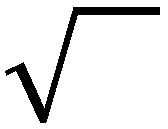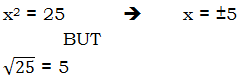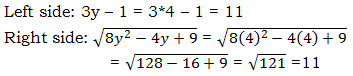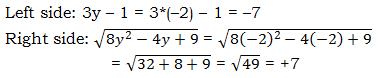This is a potentially tricky topic. First, consider these practice questions.

2x - 2 = \sqrt{3x^2 + 13}

Q1. Which value(s) of x satisfies the equation above?

I. -1

II. 4

III. 9

(A) I

(B) III

(C) I & II

(D) I & III

(E) I, II, & III

Q2. What is the value of y?

Statement #1: 3y - 1 = \sqrt{8y^2 - 4y + 9}

Statement #2: y^2 - 2y - 8 = 0

Q3. If Ed's annual salary is \${360,000} what is Rick's annual salary? (1) The sum of Rick's annual salary and Jim's annual salary is equal to Ed's annual salary. (2) The sum of Rick's annual salary and Ed's annual salary is equal to twice Jim's annual salary. A. Statement 1 ALONE is sufficient to answer the question, but statement 2 alone is NOT sufficient. B. Statement 2 ALONE is sufficient to answer the question, but statement 1 alone is NOT sufficient. C. BOTH statements 1 and 2 TOGETHER are sufficient to answer the question, but NEITHER statement ALONE is sufficient. D. Each statement ALONE is sufficient to answer the question. E. Statement 1 and 2 TOGETHER are NOT sufficient to answer the question. A full discussion of these problems will come at the end of this article. ## A subtle distinction Consider the following, very simple algebra equation x^2 = 25 Of course, this equation has two solutions, +5 and -5. Of course, we find that by taking the square-root of both sides, remembering this process involves a \pm sign. Now, by contrast, consider this expression: \sqrt{25} Many folks might think this is, in all respects, the same as the solution in the first process — i.e. "taking the square root." We need to draw a subtle distinction here, concerning the nature of this sign:The benighted unfortunately will refer to this as a "square root" sign, but that is a misleading partial name. The name of this simple is the "principal square root" sign, where the word "principal", in the sense of "main" or "primary", here means the positive root only. Accordingly, the output of this sign is always positive. The number 25 has two square roots, one positive and one negative, but it has only one principal square root. A number can have, at most, only one principal square root. The principal square root of 25 is +5 only. When a squared algebraic expression appears in a problem, and we ourselves, in the process of problem-solving, find a square root, we need to include all roots, positive and negative (a common mistake is to forget the negative roots). BUT, when the symbol above, the principle square root symbol, is printed on the page as part and parcel of the given problem, this means its output will always be positive. Thus, the paradoxical juxtaposition## Solving an equation with a radical By "equation with a radical", I will be referring to any algebraic equation in which some of the algebra is under a radical sign, a.k.a a principal square root sign. The general strategy for such an equation is (a) isolate the radical (i.e. get it alone by itself on one side of the equation); (b) square both sides, thus eliminating the radical; and (c) solve what remains using algebra (often, this will involve factoring a quadratic.) All well and good, but there's a catch. You see, when you square both sides of equation, sometimes that creates solutions that weren't part of the original equation. Consider the hyper-simple equation x = 5. This "equation" has only one solution, positive five. BUT, if we square both sides, then we get the equation we solved in the previous section, with solutions \pm 5. The extra root, x = -5, was not a solution of the original equation, but it became a solution once we squared. This is an example of an extraneous root — a number that is not a root of the original equation, but which "becomes" a root when we square both sides. We have to square both sides to solve an equation with radicals, but doing so introduces the possibility of an extraneous root. Thus, an essential part of solving any equation with radicals is to check the answers you find, in order to ascertain whether any are extraneous roots. We verify the roots by plugging them into the original equation — if the number does not solve the original equation, as given in the problem, then it is not a bona fide solution. BTW, just as a general point of strategy, regardless of whether radicals are involved, I recommend checking any algebra values you find by plugging them back into the original equation given in the problem, when possible. It's just a good habit to check your work. Extraneous roots play a role in both of the sample problems above. Having read this post, you may want to go back and give them another attempt before reading the solutions below. Questions? Let us know in the comment section at the bottom. ## Solutions to the sample questions Q1. The radical is already isolated, so square both sides. (2x - 2)^2 = 3x^2 + 13 4x^2 - 8x + 4 = 3x^2 + 13 x^2 - 8x - 9 = 0 (x - 9)(x + 1) = 0 preliminary solutions: x = \{+9, -1\} At this point, an unsuspecting student might be tempted to answer (C), the trap answer. BUT, the problem is: one or both of these answers could be extraneous. We need to check each by plugging back into the original expression. Check x = -1 left side: 2x - 2 = 2(-1) - 2 = 4 right side: \sqrt{3x^2 + 13} = \sqrt{3(9)^2 + 13} = \sqrt{243 + 13} = \sqrt{256} = 16 The value x = 9 checks — it makes the two sides equal, and thus satisfies the original equation. This is the only solution, so only option III contains a root. Answer = (B) Q2. Start with the easier statement, Statement #2. Statement #2: y^2 - 2y - 8 = 0 (y - 4)(y + 2) = 0 y = \pm 4 or y = -2 Since there are two values of y, this statement, alone and by itself, is not sufficient. Statement #1: 3y - 1 = \sqrt{8y^2 - 4y + 9} The radical is already isolated, so square both sides. (3y - 1)^2 = 8Y^2 - 4y + 9 9y^2 - 6y + 1 = 8y^2 - 4y + 9 y^2 - 2y - 8 = 0 Lo and behold! We have arrived at the same equation we found in Statement #2, with solutions y = +4 or y = -2. The naïve conclusion would be — this statement says exactly the same thing as the other. That's incorrect, though, because we don't know whether both of these values are valid solutions, or whether one or more is an extraneous root. We need to test this in the original equation. Test y = +4This value checks — y = +4 is a valid solution to the equation Test y = -2The two sides are not equal, so this does not check! This value, y = -2, is an extraneous root. Thus, the equation given in Statement #1 has only one solution, y = 4, so this equation provides a definitive answer to the prompt question. This statement, alone and by itself, is sufficient. Answer = (A) Q3. This question is dealing with systems of equations. It wants us to decide if we can determine Rick's salary based on Ed's salary (\${360,000}) and two other pieces of information, which includes the salary of a third coworker, Jim: (1) The sum of Rick's annual salary and Jim's annual salary is equal to Ed's annual salary and (2) The sum of Rick's annual salary and Ed's annual salary is equal to twice Jim's annual salary.

It's helpful to start with some variables, so let's make R = Rick's \space annual \space salary and J = Jim's \space annual \space salary.

Let's walk through the statements to see if they are sufficient for determining the value of R.

1) The sum of Rick's annual salary and Jim's annual salary is equal to Ed's annual salary

We can create an equation from this: R + J = \${360,000} But we've run into a problem. We can't solve for an equation when we have two variables, unless we know more about their values. So this is a dead-end—we can't solve for R based on 1) alone. Now let's look at 2). (2) The sum of Rick's annual salary and Ed's annual salary is equal to twice Jim's annual salary. We can create an equation from this as well: R + E = 2J When we plug in Ed's salary, we get: R + 360,000 = 2J Again, we have an equation with two variables, which puts us in another dead end. Therefore, we can't solve for R based on 2) alone. But what happens if we combine the two statements, and therefore the two equations? We end up with: R + J = 360,000 R - 2J = -360,000 Technically we don't need to do any more work here, because all we need to do is prove that we need both equations to solve for R. With a system of equations that has two variables, we need at least two equations to find a solution. We have those conditions here: 2 variables, 2 equations. So, we know for certain we can solve for R and J. The answer, therefore, is C. However, here is how we could show our work to solve for R: R + J = 360,000 R - 2J = -360,000 Multiply the first equation by 2: 2R + 2J = 720,000 R - 2J = -360,000 Add the two together. The -2J and 2J will cancel, leaving us with: 3R = 360,000 R = 120,000 Thus, we know that Rick's salary is \${120,000}. While that seems like our "answer," keep in mind that that isn't our answer --- our answer was a few steps earlier, when we determined we can solve for Rick's salary. What his salary actually is doesn't matter --- the point of the question is only to find out whether or not we can solve for it.